Share

# Frank solutions for Class 10 Chemistry chapter 8 - Study of Compounds-I: Hydrogen Chloride

#### Chapters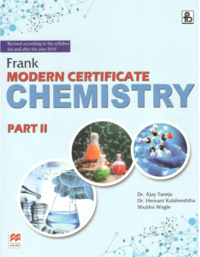## Chapter 8: Study of Compounds-I: Hydrogen Chloride

Exercise

#### Frank solutions for Class 10 Chemistry Chapter 8 Exercise [Page 198]

Exercise | Q 1.01 | Page 198

Name the following :
Acid present in the stomach of mammals.

Exercise | Q 1.02 | Page 198

Name the following:
Drying agent used to dry hydrogen chloride.

Exercise | Q 1.03 | Page 198

Name the following:
Salt obtained by heating sodium chloride with concentrated sulphuric acid below 200 degree celcius.

Exercise | Q 1.04 | Page 198

Name the following:
Gas that yields white precipitates dense white fumes when mixed with hydrogen chloride.

Exercise | Q 1.05 | Page 198

Name the following:
Two solutions that yields white precipitates, when treated with hydrogen chloride or hydrochloric acid.

Exercise | Q 1.06 | Page 198

Name the following:
Gas obtained by treating metals with hydrochloric acid.

Exercise | Q 1.07 | Page 198

Name the following:
Gas obtained by treating ferrous sulphide with hydrochloric acid.

Exercise | Q 1.08 | Page 198

Name the following:
Five oxidizing agents that liberated chlorine from concentrated hydrochloric acid.

Exercise | Q 1.09 | Page 198

Name the following:
Acid used to extract glue from bones.

Exercise | Q 1.1 | Page 198

Name the following:
A chloride which is soluble in excess of ammonium hydroxide.

Exercise | Q 1.11 | Page 198

Name the following:  A greenish yellow gas.

Exercise | Q 1.12 | Page 198

Name the following:
A chemical in which gold can be dissolved.

Exercise | Q 1.13 | Page 198

Name the following:
A metallic oxide which reacts with hydrochloric acid to give a coloured solution.

Exercise | Q 1.14 | Page 198

Name the following:
Two colourless gases which when mixed produce a white solid.

#### Frank solutions for Class 10 Chemistry Chapter 8 Exercise [Pages 198 - 200]

Exercise | Q 1 | Page 199

Calcium oxide and phosphorous pentoxide are very good drying agents but they are not used to dry HCI gas. Give reasons for each.

Exercise | Q 2.1 | Page 198

Draw a labelled diagram and explain the laboratory preparation of hydrogen chioride gas.

Exercise | Q 2.2 | Page 199

How will you prove that the gas prepared is HCI?

Exercise | Q 2.3 | Page 200

What is the function of HCI in preparation of aqua-regia?

Exercise | Q 2.3 | Page 199

Name the drying agent used to dry HCl gas.

Exercise | Q 2.4 | Page 199

How is the gas collected and why?

Exercise | Q 2.5 | Page 199

What are the important precautions?

Exercise | Q 3 | Page 199

How will you prepare hydrochloric acid in laboratory? OR
Describe briefly the method employed to dissolve hydrogen chloride gas in water as it is prepared. What is the purpose of funnel while preparing hydrochloric acid from HCI gas?

Exercise | Q 4.1 | Page 199

Explain, why (or give reasons for)
In the preparation chloride from sodium chloride, the gas can be obtained below 200°C or above. But the lower temperature is preferred.

Exercise | Q 4.2 | Page 199

Explain, why (or give reasons for)
Hydrogen chloride is not collected over water.

Exercise | Q 4.3 | Page 199

Explain, why (or give reasons for)
Hydrochloric acid cannot be concentrated above 22.2% by boiling.

Exercise | Q 4.4 | Page 199

Explain, why (or give reasons for)
HCI gas does not conduct electricity, but hydrochloric acid conducts electricity.

Exercise | Q 4.5 | Page 199

Explain, why (or give reasons for)
Only a very dilute solution of hydrogen chloride in water can be concentrated by boiling the solution while a very concentrated solution would become less concentrated when boiled.

Exercise | Q 4.6 | Page 199

Explain why When the stopper of a bottle full of hydrogen chloride gas is opened there are fumes in the air.

Exercise | Q 4.7 | Page 199

Explain, why (or give reasons for)
A solution of HCl gas in water turns blue litmus red and conducts electricity, while HCI gas dissolves in toluene and it has no effect on litmus and does not conduct electricity.

Exercise | Q 4.8 | Page 199

Explain, why (or give reasons for)
An aqeous solution of chlorine is acidic is nature.

Exercise | Q 5.1 | Page 199

What would happen if
Calcium oxide is used to dry hydrogen chloride.

Exercise | Q 5.2 | Page 199

What would happen if :
Concentrated hydrochloric acid is kept open.

Exercise | Q 5.3 | Page 199

What would happen if
Hydrogen chloride prepared in laboratory, is passed through water, using a delivery tube.

Exercise | Q 6 | Page 199

Write three equations to show that hydrochloric acid acts as an acid.

Exercise | Q 7.1 | Page 199

Describe an experiment to prove the following:
HCI gas is heavier than air.

Exercise | Q 7.2 | Page 199

Describe an experiment to prove the following:
HCI is highly soluble in water.

Exercise | Q 7.3 | Page 199

Describe an experiment to prove the following:
HCI gas contains the element chlorine.

#### Frank solutions for Class 10 Chemistry Chapter 8 Exercise [Page 200]

Exercise | Q 1.1 | Page 200

Name the gas evolved when dilute hydrochloric acid is added to: Potassium bisulphite

Exercise | Q 1.1 | Page 200

Name the gas evolved when dilute hydrochloric acid is added to: Calcium carbonate

Exercise | Q 1.1 | Page 200

Name the gas evolved when dilute hydrochloric acid is added to:  Sodium sulphite

Exercise | Q 1.1 | Page 200

Name the gas evolved when dilute hydrochloric acid is added to: Lead (II) sulphide

Exercise | Q 1.1 | Page 200

Name the gas evolved when dilute hydrochloric acid is added to: Magnesium bicarbonate

Exercise | Q 1.1 | Page 200

Name the gas evolved when dilute hydrochloric acid is added to: Zinc Metal

Exercise | Q 1.2 | Page 200

Give a balanced equation when dilute hydrochloric acid is added to : Potassium bisulphite

Exercise | Q 1.2 | Page 200

Give a balanced equation when dilute hydrochloric acid is added to : Calcium carbonate

Exercise | Q 1.2 | Page 200

Give a balanced equation when dilute hydrochloric acid is added to : Sodium sulphite

Exercise | Q 1.2 | Page 200

Give a balanced equation when dilute hydrochloric acid is added to : Lead (II) sulphide

Exercise | Q 1.2 | Page 200

Give a balanced equation when dilute hydrochloric acid is added to : Magnesium bicarbonate

Exercise | Q 1.2 | Page 200

Give a balanced equation when dilute hydrochloric acid is added to :  Zinc Metal

Exercise | Q 2.1 | Page 200

What is aqua-regia?

Exercise | Q 2.2 | Page 200

State the use of aqua-regia.

Exercise | Q 3 | Page 200

Give two tests for hydrochloric acid.

Exercise | Q 4 | Page 200

Write the uses of hydrochIoric acid.

Exercise | Q 5.1 | Page 200

Mention the reaction condition and give balanced equation to obtain: Cl2 gas from HCI gas.

Exercise | Q 5.2 | Page 200

Mention the reaction condition and give balanced equation to obtain: Cl2 gas from HCI acid.

Exercise | Q 5.3 | Page 200

Mention the reaction condition and give balanced equation to obtain : HCl gas from common salt

Exercise | Q 6.1 | Page 200

How can you prove that hydrochloric acid contain :
Hydrogen

Exercise | Q 6.2 | Page 200

How can you prove that hydrochloric acid contain :
Chlorine?

Exercise | Q 7.1 | Page 200

Fill in the blank:
Aqua-regia is a mixture of ___________ .

Exercise | Q 7.2 | Page 200

Fill in the blank:
A constant boiling mixture of water and hydrochloric acid is also called as _______________.

Exercise | Q 7.3 | Page 200

Fill in the blank:
___________ agent converts hydrochloric acid to chlorine.

Exercise | Q 7.4 | Page 200

Fill in the blank:
Hydrogen and chlorine reacts in presence of ___________ to form hydrogen chloride.

Exercise | Q 7.5 | Page 200

Fill in the blank:
On addition of silver nitrate to hydrochloric acid ___________ precipitate is formed which is soluble in  ____________

Exercise | Q 7.6 | Page 200

Fill in the blank:
The white precipitate of lead chloride is soluble in _________

Exercise | Q 8.1 | Page 200

Choose the correct answer from the options given below: HCl gas can be prepared by direct combination of hydrogen and chlorine gas in presence of

• Direct sunlight

• Dark atmosphere

• Diffused sunlight

• MnO2 catalyst

Exercise | Q 8.2 | Page 200

Choose the correct answer from the options given below:
Dilute hydrochloric acid solution cannot be concentrated by boiling beyond

• 11%

• 33%

• 44%

• 22%

Exercise | Q 8.3 | Page 200

Choose the correct answer from the options given below:
Bleaching powder reacts with few drops of concentrated HCl to give

• Chlorine

• Calcium oxide

• Oxygen

• None of these

Exercise | Q 8.4 | Page 200

Choose the correct answer from the options given below:
Which of the following statement is not correct ?

• HCl gas is collected by upward displacement of air

• HCl acid gives white precipitate with AgNO3.

• HCl gas is collected by downward displacement of air

• HCl acid turns phenolphthalein solution colurless

#### Frank solutions for Class 10 Chemistry Chapter 8 Exercise [Page 201]

Exercise | Q 1 | Page 201

Explain, why silver nitrate crystals are dissolved in distilled water and not in tap to prepare silver nitrate solution as a laboratory reagent.
Give one chemical test to distinguish between dilute hydrochloric acid and dilute sulphuric acid.

Exercise | Q 2.1 | Page 201

Name two gases which combine chemically to form a solid.

Exercise | Q 2.2 | Page 201

Name a chloride which is soluble in excess of ammonium hydroxide.

Exercise | Q 2.3 | Page 201

How will you show that hychloric acid contains both hydrogen and chlorine (other than by electrolysis) ?

Exercise | Q 2.4 | Page 201

Complete and balance the equations:
KMnO4 + HCI → _______+ _______ + _______ + ______ + 8H2O

Exercise | Q 3 | Page 201

Hydrogen chloride gas is very soluble in water. It is dissolved in water to prepare hydrochloric acid by using an anti-suction device, as shown in the diagram. Very briefly explain how this device prevents the suction of water into the flask, in which hydrogen chloride gas is produced.

Exercise | Q 4 | Page 201

Give reason for the following:
Dilute hydrochloric acid cannot be concentrated by distilling (boiling) the dilute acid.

Exercise | Q 5 | Page 201

Dilute hydrochloric acid is added in turn to a mixture of iron and sulphur and to the compound formed between iron and sulphur. Name the gas formed in each case.

Exercise | Q 6 | Page 201

Name the gas evolved when an oxide and concentrated hydrochloric acid are heated.

Exercise | Q 7 | Page 201

Explain, why the following statement is not correct:

Exercise | Q 8 | Page 201

Outline the steps required to convert hydrogen chloride to anhydrous FeCl3. Write equations for the equations involved.

#### Frank solutions for Class 10 Chemistry Chapter 8 Exercise [Page 202]

Exercise | Q 1 | Page 202

State what you see, when siIver nitrate solution is added to dilute HCl.

Exercise | Q 2.1 | Page 202

What must be added to sodium chloride to prepare hydrogen chloride?

Exercise | Q 2.2 | Page 202

Write the equation for the reaction which takes place in question(a).

Exercise | Q 2.3 | Page 202

What will you observe, when hydrogen chlorides mixed with ammonia?

Exercise | Q 3.1 | Page 202

Hydrogen chloride dissolves in water to form an acidic solution.
Name the experiment which demonstrates that hydrogen chloride is very soluble in water.

Exercise | Q 3.2 | Page 202

Hydrogen chloride dissolves in water to form an acidic solution.
Give three distinct tests (apart from using an indicator) you would carry out with this solution to illustrate the typical properties of an acid.

Exercise | Q 4.1 | Page 202

Write equation for the reaction between hydrochloric acid of the following: Bleaching powder

Exercise | Q 4.2 | Page 202

Write equation for the reaction between hydrochloric acid of the following: Lead nitrate solution

Exercise | Q 4.3 | Page 202

Write equation for the reaction between hydrochloric acid of the following: Manganese dioxide

Exercise | Q 4.4 | Page 202

Write equation for the reaction between hydrochloric acid of the following: Oxide of lead

Exercise | Q 5 | Page 202

What wilI you observed when concentrated HCI is added to lead (IV) oxide and warmed ?

Exercise | Q 6 | Page 202

Write balanced equation for the reaction between dilute hydrochloric acid and sodium sulphite.

Exercise | Q 7.1 | Page 202

Write the equation for :
The preparation of hydrogen chloride from sodium chloride and sulphuric acid. State whether the sulphuric acid should be concentrated or dilute.

Exercise | Q 7.2 | Page 202

Write the equation for:
The reaction between hydrogen chloride and ammonia.

Exercise | Q 8 | Page 202

Name one lead compound that can be used to oxidize hydrogen chioride to chIorine.

Exercise | Q 9 | Page 202

What happens when dilute hydrochloric acid is added to Iead nitrate solution ?

Exercise | Q 10 | Page 202

What happens when dilute hydrochloric acid is added to Iead nitrate solution ?

Exercise | Q 11 | Page 202

Write balanced equation for the following reaction:

Exercise | Q 12 | Page 202

A solution of hydrogen chloride in water is prepared. The following substances are added to separate portions of the solution:

 S.No. Substance added Gas Evolved Odour 1 Calcium carbonate ______ ______ 2 Magnesium ribbon ______ ______ 3 Manganese (IV) oxide with heating ______ ______ 4 Sodium Sulphide _______ _______

Complete the table by writing the gas evolved in each case and its odour.

#### Frank solutions for Class 10 Chemistry Chapter 8 Exercise [Page 203]

Exercise | Q 1 | Page 203

State one reason why tap water is not used to prepare a solution of silver nitrate in the laboratory.

Exercise | Q 2.1 | Page 203

Write balanced equation for the following reaction:
Copper oxide and dilute hydrochloric acid.

Exercise | Q 2.2 | Page 203

Write balanced equcation for the following reaction:
Manganese (IV) oxide and concentrated hydrochloric acid.

Exercise | Q 3 | Page 203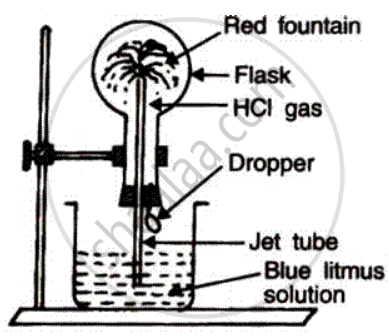(a) Name the experiment illustrate above.
(b) Which property of hydrogen chloride is demonstrated by this experiment?
(c) State the colour of the water that has entered the round-bottomed flask.

Exercise | Q 4.1 | Page 203

Write a balanced equation for the reaction of zinc and dilute hydrochloric acid.

Exercise | Q 4.2 | Page 203

State what is observed when hydrochloric acid is added to silver nitrated solution.

Exercise | Q 5.1 | Page 203

of the two gases ammonia and hydrogen chloride, which is more dense. Name the method of collection of this gas.

Exercise | Q 5.2 | Page 203

Give one example of a reaction between the ammonia and hydrogen chloride gases which produces a solid
compound.

Exercise | Q 6.1 | Page 203

What is property of concentrated sulphuhric acid which allows it to be used in the preparation of hydrogen chloride and nitric acid?

Exercise | Q 6.2 | Page 203

What property of hydrogen chloride is demonstrated when it is collected by downward delivery (upward displacement) ?

Exercise | Q 6.3 | Page 203

Why is hydrogen chloride not collected over water?

Exercise | Q 7.1 | Page 203

Write a fully balanced of the following case :
Red lead is warmed with concentrated hydrochloric acid.

Exercise | Q 7.2 | Page 203

Write a fully balanced of the following case :
Magnesium metal is treated with dilute hydrochloric acid.

Exercise | Q 8 | Page 203

Correct the following statement:
Hydrochloric acid is prepared in the laboratory by passing hydrogen chloride directly through water.

Exercise | Q 9 | Page 203

Choose the correct answer from the option given below:
Aqua regia is a mixture of :

• Dilute hydrochloric acid and concentrated nitric acid

• Concentrated hydrochloric acid and dilute nitric acid

• Cocentrated hydrochloric acid [1 part] and concentrated nitric acid [ 3 parts]

• Concentrated hydrochloric acid [3 parts] and concentrated nitric acid [1 part]

#### Frank solutions for Class 10 Chemistry Chapter 8 Exercise [Page 204]

Exercise | Q 1 | Page 204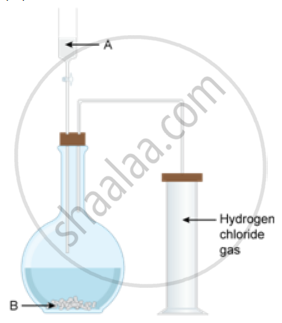The diagram shows an apparatus for the laboratory preparation of hydrogen chloride.
(i)
Identify A and B
(ii) Write the equation for the reaction
(iii) How would you check whether or not the gas jar is filled with hydrogen chloride?
(iv) What does the method of collection tell you about the density of hydrogen chloride.

Exercise | Q 2 | Page 204

By the addition of only one solution how would you distinguish between dilute hydrochloric acid and dilute nitric acid?

Exercise | Q 3 | Page 204

Choose the correct answer from the options given below :
Hydrogen chloride gas being highly soluble in water is dried by :

• Anhydrous calcium chloride

• Phosphorous pentaoxide

• Quick time

• Concentrated sulphuric acid

Exercise | Q 4.1 | Page 204

In the laboratory preparation of hydrochloric acid, HCl gas is dissolved in water.
Draw a diagram to show the arrangement used for the absorption of HCl in water.

Exercise | Q 4.2 | Page 204

In the laboratory preparation of hydrochloric acid,HCl gas is dissolved in water.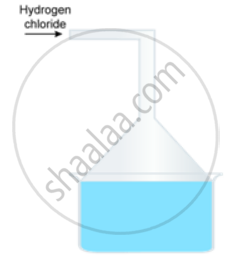Why is such an arrangement necessary? Give two reasons.

Exercise | Q 4.3 | Page 204

In the laboratory preparation of hydrochloric acid, HCl gas is dissolved in water.
Write the chemical equation for the laboratory preparation of HCl gas when the reactants are :
(a) Below 200oC
(b) Above 200oC

Exercise | Q 5.1 | Page 204

State one appropriate observation  of the following :
Copper sulphate is treated with dilute hydrochloric acid

Exercise | Q 5.2 | Page 204

State one appropriate observation of the following:
A few drops of dilute hydrochloric acid are added to silver nitrate solution, followed by the addition of ammonium hydroxide solution.

Exercise | Q 5.3 | Page 204

State one appropriate observation of the following:
Which gas is evolved when potassium sulphite with dilute hydrochloric acid.

Exercise | Q 5.4 | Page 204

State one appropriate observation of the following:
Concentrated HCl is made to react with mangesediaoxide

Exercise | Q 5.5 | Page 204

State one appropriate observation of the following:
Action of dilute HCl or sodium sulphite.

Exercise | Q 6 | Page 204

Study the figure given below and answer that questions that follow: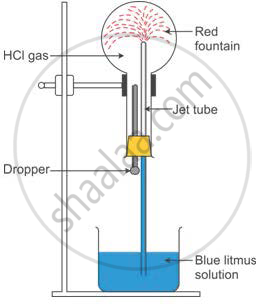(i) Identify the gas Y.
(ii) What property of gas Y does this experiment demonstrate?
(iii) Name another gas which has the same property and can be demonstrated through this experiment.

#### Frank solutions for Class 10 Chemistry Chapter 8 Exercise [Page 205]

Exercise | Q 1.1 | Page 205

The following question is pertaining to the laboratory pertaining hydrogen chloride gas.
Write the equation for its preparation mentioning the condition required

Exercise | Q 1.2 | Page 205

The following question is pertaining to the laboratory pertaining hydrogen chloride gas.
Name the drying agent used and justify your choice

#### Frank solutions for Class 10 Chemistry Chapter 8 Exercise [Page 205]

Exercise | Q 1.3 | Page 205

The following question is pertaining to the laboratory pertaining hydrogen chloride gas.
State a safety precaution you would take during the preparation of hydrochloric acid.

Exercise | Q 2 | Page 205

The aim of the fountain experiment is to prove that

• HCl turns blue litmus red

• HCl is denser than air

• HCl is highly soluble in water

• HCl fumes in moist air

Exercise | Q 3.1 | Page 205

Dilute hydrochloric acid is added to lead nitrate solution and the mixture is heated

Exercise | Q 3.2 | Page 205

Dilute hydrochloric acid is added to sodium thisulphate

Exercise | Q 3.3 | Page 205

Dilute hydrochloric acid is added to copper carbonate.

Exercise | Q 4 | Page 205

State the observation for action of dilute hydrochloiric acid or iron (II) sulphate.

Exercise | Q 5 | Page 205

How will you distinguished between dilute HCl and dilute H2SO4 using lead nitrate solution?

## Chapter 8: Study of Compounds-I: Hydrogen Chloride

Exercise## Frank solutions for Class 10 Chemistry chapter 8 - Study of Compounds-I: Hydrogen Chloride

Frank solutions for Class 10 Chemistry chapter 8 (Study of Compounds-I: Hydrogen Chloride) include all questions with solution and detail explanation. This will clear students doubts about any question and improve application skills while preparing for board exams. The detailed, step-by-step solutions will help you understand the concepts better and clear your confusions, if any. Shaalaa.com has the CISCE Frank Class 10 Chemistry Part 2 solutions in a manner that help students grasp basic concepts better and faster.

Further, we at Shaalaa.com provide such solutions so that students can prepare for written exams. Frank textbook solutions can be a core help for self-study and acts as a perfect self-help guidance for students.

Concepts covered in Class 10 Chemistry chapter 8 Study of Compounds-I: Hydrogen Chloride are Hydrogen Chloride - Acidic Properties of Its Solution, Hydrogen Chloride - Reaction with Ammonia, Hydrogen Chloride - Density and Solubility of Hydrogen Chloride (Fountain Experiment), Hydrogen Chloride - Preparation of Hydrogen Chloride from Sodium Chloride, Concept of Hydrogen Chloride, Method of Preparation of Hydrochloric Acid, Precipitation Reactions with Silver Nitrate Solution and Lead Nitrate Solution.

Using Frank Class 10 solutions Study of Compounds-I: Hydrogen Chloride exercise by students are an easy way to prepare for the exams, as they involve solutions arranged chapter-wise also page wise. The questions involved in Frank Solutions are important questions that can be asked in the final exam. Maximum students of CISCE Class 10 prefer Frank Textbook Solutions to score more in exam.

Get the free view of chapter 8 Study of Compounds-I: Hydrogen Chloride Class 10 extra questions for Chemistry and can use Shaalaa.com to keep it handy for your exam preparation

S